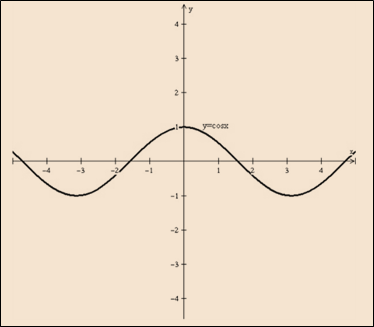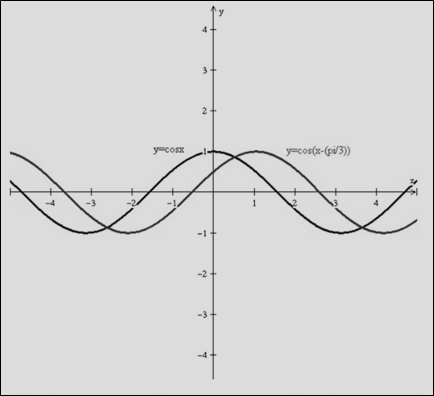# To graph: The following function with the help of the transformation: y = cos ( x − π 3 )### Single Variable Calculus: Concepts...

4th Edition
James Stewart
Publisher: Cengage Learning
ISBN: 9781337687805### Single Variable Calculus: Concepts...

4th Edition
James Stewart
Publisher: Cengage Learning
ISBN: 9781337687805

#### Solutions

Chapter C, Problem 37E
To determine

## To graph: The following function with the help of the transformation:   y=cos(x−π3)

Expert Solution

### Explanation of Solution

Given information:

y=cos(xπ3)

Graph:Graph 1: y=cosxGraph 2: y=cos(xπ3)

Interpretation:

Need to draw a first graph of the function y=cosx as shown by the graph 1. Now, the given function y=cos(xπ3) is in form of the y=f(xc) . It means that need to shift the graph by y=f(x)c on the right side.

Hence, the graph shifted towards right y=cos(xπ3) with the transformation and it shown by the graph 2.

### Have a homework question?

Subscribe to bartleby learn! Ask subject matter experts 30 homework questions each month. Plus, you’ll have access to millions of step-by-step textbook answers!